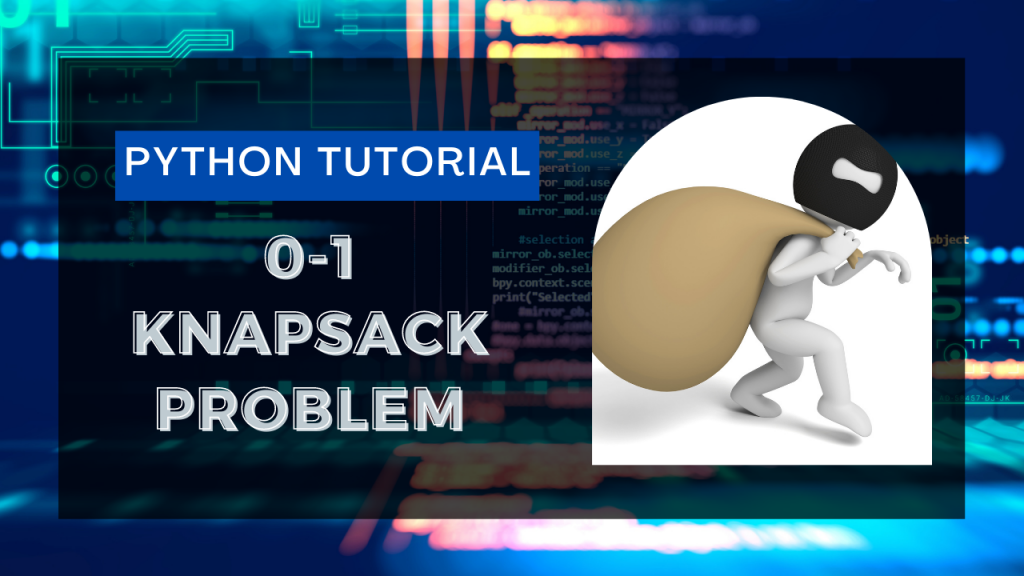# Solving the 0-1 Knapsack Problem in Python using RecursionHi guys! In this tutorial, I am trying to explain the knapsack problem. You will encounter the problem somewhere or another during the interviews.

We will be using the Recursion approach in order to solve the problem. If you are unaware of how recursion works take a look at the tutorial below.

## Introduction to Knapsack Problem

There’s a thief with a knapsack that can hold a total weight of `capacity`. He has n items to steal from the place having different weights and prices.

Our aim is to create a function called `knapsack` that will find out a subset of these items resulting in the maximum profit for the thief considering the total weight of all the items does not exceed the given `capacity` of the knapsack.

The same is illustrated in the image below.

## Solution to the Knapsack Problem in Python using Recursion

We will be considering that for each item the thief has two options: Either to include the item or to exclude the item and don’t pick it up.

If the thief includes an item, we will be searching for maximum profit for the remaining n-1 items and will also decrease the capacity by the weight of the item included.

The total profit, in this case, would be: the price of item + Profit in n-1 items for (capacity – the weight of the item) remaining

And if a person excludes the item, we will just find the profit for the remaining n-1 items from the store. The profit, in this case, will be: Profit in n-1 items for capacity remaining

The final answer would be the maximum profit from both cases.

## Code Implementation

```def knapsack(n,capacity,weights,prices):
if(n==0 or capacity==0):
return 0

if (weights[n-1]>capacity):
return knapsack(n-1,capacity,weights,prices)

else:
return max(prices[n-1] + knapsack(n-1,capacity-weights[n-1],weights,prices),
knapsack(n-1,capacity,weights,prices))

weights = [1,2,3,4]
prices = [50,200,150,100]
n = 4
capacity = 7

print(knapsack(n,capacity,weights,prices))
```

The output received after the execution of the current code came out to be 400 which is the correct output.

Thank you for taking the time for reading the tutorial! I hope the Knapsack problem is clear to you now.

Happy learning! 😇